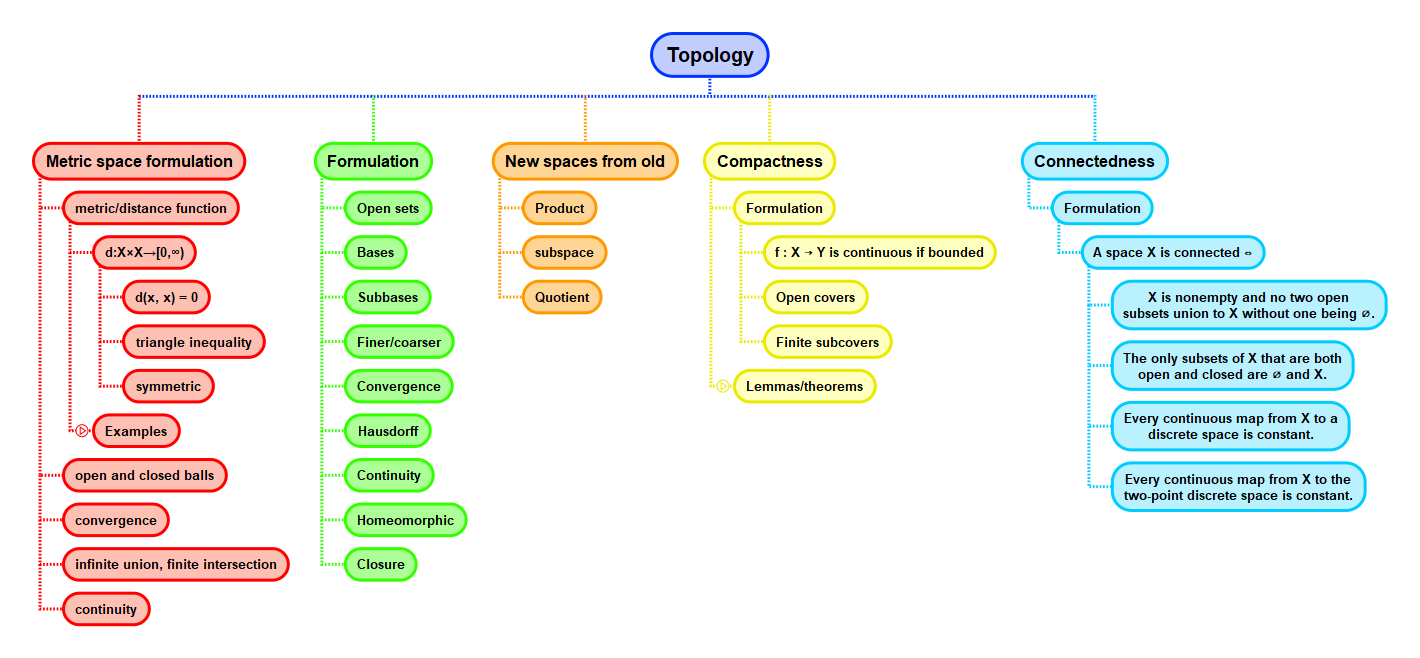Show Question
Math and science::Topology

# Basis

Instead of specifying all possible open sets of a topology, it is convenient to be able to specify the topology in terms of a smaller set. Analogously, for metric spaces, the set of open balls could be used to describe a metric space instead of directly specifying the arbitrary open sets. For topological spaces, a basis carries out this role (plural: bases).

### Basis, definition

Let $$X$$ be a topological space. A basis for $$X$$ is a collection $$\mathscr{B}$$ of open subsets of $$X$$ such that every open subset of $$X$$ is a union of sets in $$\mathscr{B}$$.

Two consequence of this definition are:

• Every element of $$X$$ has at least one neighbourhood that is a basis element.
• the intersection of any two basis elements must be a basis element.

A bit more formally, these two consequences translate to:

### Lemma.

Let $$X$$ be a topological space, and let $$\mathscr{B}$$ be a basis for $$X$$. Then:

1. For each $$x \in X$$, there is at least one basis element $$B$$ containing $$x$$.
2. If $$x \in B_1 \cap B_2$$ where $$B_1$$ and $$B_2$$ are basis elements, then $$x \in B_3$$ for some basis element $$B_3 \subseteq B_1 \cap B_2$$.

#### The choice of definition and lemma

The wording of this lemma is presented in line with Munkres's presentation. Munkres, however, presents this lemma as the definition of a basis; he then proceeds to derive the content of the definition that I've presented above. Leinster proceeds in the opposite order, as presented here. I think that the motivation of a basis is clearer from Leinster's definition, whereas the lemma presented by Leinster (definition for Munkres) is more useful to work with. I followed Munkres's wording for the lemma as I feel it encourages thinking in terms of individual elements of $$X$$ which so far has been useful in proofs.

## Context